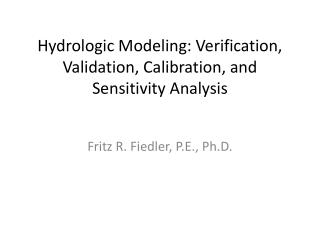# Hydrologic Modeling: Verification, Validation, Calibration, and Sensitivity Analysis - PowerPoint PPT PresentationDownload PresentationHydrologic Modeling: Verification, Validation, Calibration, and Sensitivity Analysis

Presentation Description
Download Presentation## Hydrologic Modeling: Verification, Validation, Calibration, and Sensitivity Analysis

- - - - - - - - - - - - - - - - - - - - - - - - - - - E N D - - - - - - - - - - - - - - - - - - - - - - - - - - -
##### Presentation Transcript

1. Hydrologic Modeling: Verification, Validation, Calibration, and Sensitivity Analysis Fritz R. Fiedler, P.E., Ph.D.

2. Definitions (review) • Verification: check if code solves equations correctly • Validation: check if model reasonably represents physical process • Calibration: adjust model parameters to match observations • Sensitivity Analysis: relative effect of parameter changes on output

3. Verification • Compare numerical results to analytical results

4. Level 1 Validation • Compare model results to simple experiments (can estimate parameters a priori)

5. Calibration • Adjust parameters to match observations

6. Level 2 Validation • Compare model results to observations for a different input data set post-calibration • Reserve some data (do not use in calibration) • After finding parameters that result in “best fit,” run model with reserved input and compare to output • Problems with this? • What happens in practice?

7. Sensitivity Analysis • Explore how parameter changes affect output • Sensitivity index:

8. Calibration Targets Can physically based model parameters be measured? Why or why not?

9. Goodness of Fit • Visual comparison between simulated and observed – look for trends in errors • A learned art • Use appropriate graph scales • Statistical performance measures • Consider mean daily discharge as calibration target • Q = observed • S = simulated

10. Means and Bias Common calibration strategy: fix bias first, revisit periodically, goal of no bias

11. Maximum Error: • Percent Average Absolute Error

12. Sum of Squares of Errors • Most common basis for statistical goodness of fit • e.g., least squares regression, seek to minimize

13. Root Mean Squared Error • Size of error usually related to size of events or values, thus RMSE typically smaller for dry periods, small watersheds (for example) • How would you modify RMSE to facilitate comparison?

14. Percent RMSE • Normalize RMSE by mean observed • Because the magnitude of RMSE varies with magnitude of values, by minimizing RMSE only, which part of hydrographs are primarily best fit in calibration? • How can this tendency be addressed?

15. Nash-Sutcliffe • Very popular method of evaluating calibration • Reading: McCuen, R. H., Evaluation of the Nash—Sutcliffe efficiency index, Journal of Hydrologic Engineering, 11(6), 597-602, 2006 (note: author uses different variables)

16. Line of Best Fit Analyze as in regression: hypothesis testing on A and B, residual analysis, correlation coefficient…

17. Line of Best Fit – Correlation Coefficient

18. How to Use Statistical Measures • For a given time period, e.g., 1 year, and/or averages over multiple years • Look for seasonal trends

19. How to Use Statistical Measures • By flow interval (value interval) • Errors as f(Q) – aim for no systematic variation • How would you pick the intervals?

20. Exceedance Plots x x x x Q, S x x x x x x 0 percent days exceeded 100

21. Generalized Calibration Strategies • Set realistic parameter bounds before starting • Fix insensitive parameters first; focus on most sensitive • Eliminate most bias early in process, revisit • Use regionalized variables as appropriate • Combine manual and automatic techniques

22. Equifinality • Multiple combinations of parameters can lead to similar results • Issue with both multi-parameter lumped models (e.g., SAC-SMA) and spatially distributed models (e.g., CASC-2D) • Reading: Ebel, B. A. and K. Loague, Physics-based hydrologic-response simulation: Seeing through the fog of equifinality, Hydrological Processes, 20(13), 2887–2900, 2006